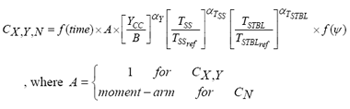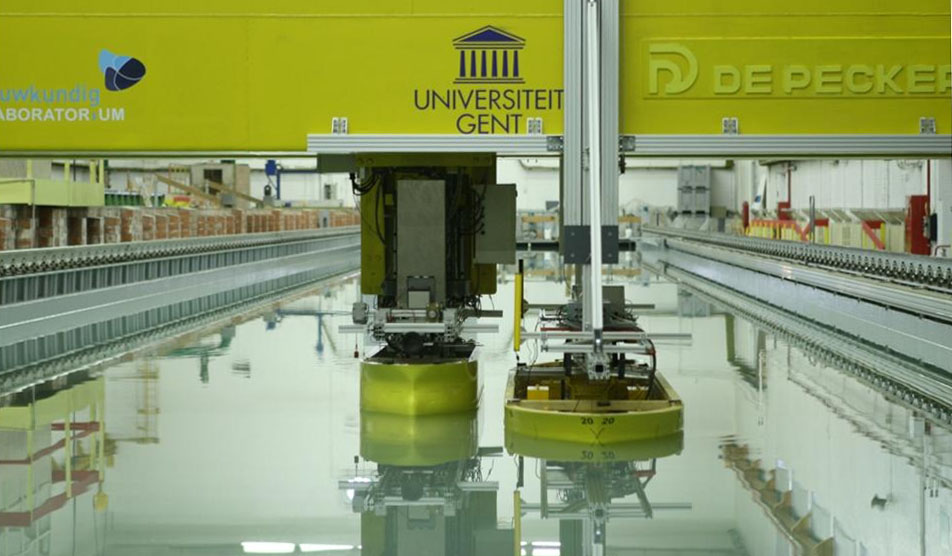Mathematical Models This work package will investigate ways of representing interaction forces and moments in fast and real time simulation models. Some existing models are using tables where forces and moments depend on the relative longitudinal and transverse positions of the vessels as found for some standard vessels. The outcome from this project will be interaction forces and moments calculated from the actual ship’s geometries, relative positions and speeds. Results from WP 1 and WP 2 will be used when developing interaction models. A review of existing interaction models will be completed late 2007. Proposals for new ways of representing interaction forces and moments will be studied in 2008. By end of 2008 subject matter experts will propose how interaction forces and moments shall be represented in the real time simulation model used by project partners. Experience from using this model will be reviewed before the end of the project with the goal of improving the model based on new results from WP 1 and WP 2 and feedback from nautical experts on ship-to-ship type operations. In earlier work done by NTNU students, it was assumed that the growth of force parameters follow a simple power-law. The general form of the equations for force and moment coefficients may then be expressed as:The coefficients, α has been allocated subscripts suitable for the considered parameter.  TSSref  and  TSTBLref are reference draughts for SS and STBL, respectively. To support development of improved mathematical models the project has prepared a test case for lightering operations. For this case model tests have been performed using the advanced Planar Motion Carriage of Flanders Hydraulics Research. Models for a VLCC and an Aframax were used and the tests were completed in August 2008Published May 4, 2007